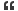# Thread: Find the Weight of Potatoes

1. ## Find the Weight of Potatoes

100 Kg. Potatoes with 98% water content are dried in an oven. The percentage of water decreases to 50 %. What is the weight of potatoes now.Reply With Quote

2. ## Re: Find the Weight of Potatoes

Around 50 kgReply With Quote

3. ## Re: Find the Weight of Potatoes

51 Percentage will be the correct one.Reply With Quote

4. ## Re: Find the Weight of Potatoes

can any one explain the answer with stepsReply With Quote

5. ## Re: Find the Weight of Potatoes

51 kg be the weight of potatoReply With Quote

6. ## Re: Find the Weight of Potatoes

New weight = 50Reply With Quote

7. ## Re: Find the Weight of Potatoes

I think 51 KgReply With Quote

8. ## Re: Find the Weight of PotatoesOriginally Posted by deepikastalincan any one explain the answer with steps
100 kg of potato caontains 98% water so wgt of water is 98kgs....
when the content of water reduces to 50% so new wgt of water is 49kgs...
so the ans is 49+ 2(100-98<the non water content>)=51Reply With Quote

9. ## Re: Find the Weight of Potatoes

It will be 51 Kg.Reply With Quote

10. ## Re: Find the Weight of Potatoes

Originally, out of 100 kg, 2 kg was solid stuff and 98 kg was liquid. After drying, 2 kg of solid stuff remains as it is and liquid stuff is reduced to 2 kg of equivalent liquid. Then, the percentage of water decreases to 50%. So the weight of potato now is 4 kg.Reply With Quote

11.

####Posting Permissions

• You may not post new threads
• You may not post replies
• You may not post attachments
• You may not edit your posts
•